## Example Questions

### Example Question #1 : How To Find The Length Of The Diameter

The formula to find the radius of the largest circle that can fit in an equilateral triangle is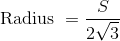, where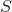is the length of any one side of the triange.

What is the largest diameter of a circle that can fit inside an equilateral triangle with a perimeter ofcm?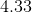cm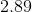cm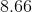cm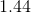cmcm

Explanation:

The diameter is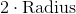To solve for the largest diameter multiply each side by 2.

The resulting formula for diamenter is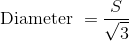.

Substitute in 5  for S and solve. Diameter =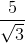= 2.89 cm

### Example Question #2 : How To Find The Length Of The Diameter

Quantity A: The diameter of a circle with area of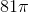Quantity B: The diameter of a circle with circumference of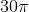Which of the following is true?

Quantity B is larger.

The relationship of the quantities cannot be determined.

Quantity A is larger.

Both quantities are equal.

Quantity B is larger.

Explanation:

Consider each quantity separately.

Quantity A

Recall that the area of a circle is defined as:We know that the area is. Therefore,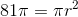Divide both sides by: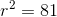Therefore,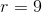. Since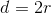, we know: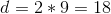Quantity B

This is very easy. Recall that: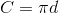Therefore, if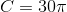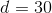. Therefore, Quantity B is larger.

### Example Question #3 : How To Find The Length Of The Diameter

Quantity A: The diameter of a circle with area of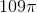Quantity B: The diameter of a circle with circumference of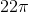Which of the following is true?

The relationship between the quantities cannot be determined.

The two quantities are equal.

Quantity A is larger.

Quantity B is larger.

Quantity B is larger.

Explanation:

Consider each quantity separately.

Quantity A

Recall that the area of a circle is defined as:We know that the area is. Therefore,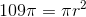Divide both sides by: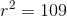Therefore,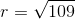.  Since, we know: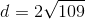Quantity B

This is very easy.  Recall that:Therefore, if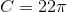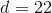.

Now, since your calculator will not have a square root button on it, we need to estimate for Quantity A. We know that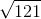is. Therefore,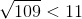.  This means that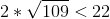. Therefore, Quantity B is larger.

Tired of practice problems?

Try live online GRE Math prep today.